×

Search anything:

# Rust for Beginners (variables, datatypes, loops, functions, array and more)

#### Rust Programming Software EngineeringIndian Technical Authorship Contest starts on 1st July 2023. Stay tuned.

Let's go through some of the basic programming concepts in Rust, such as variables, functions, loops and the like. I realize that I have not covered them in any article before, assuming some small previous knowledge.. Let's fix that by covering them today!

1. Variables & Data Types
2. Loops & Flow control
3. Functions
5. Arrays, Tuples and Vectors
6. Strings
7. Type inference and casting

# Variables & Data Types

As we've seen before, variables are declared with the let keyword, and if they need to be modified, the mut modifier is added.

We're only allowed to change the value of 'y' because it is declared as mut, which stands for mutable. If we didn't specify it's mutable, the code would not compile!
Constants on the other hand are declared with the const keyword. Naming convention is all caps with underscores between the words. Constants of course cannot be modified.

``````    const RESULT_WILL_BE: i16 = 10;
let x = 5;
let mut y = 7;
println!("The sum before change is {}", x + y);
y = 5;

println!("The sum after change is {}", x + y);
``````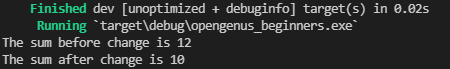In terms of data types, Rust is a statically typed language, meaning it must know all the types at compile time. Every value has a data type, otherwise the compiler doesn't know how to deal with it.

Scalar types represent a single value. There are 4 types of scalar type in Rust. Integers, Floating point numbers, booleans and characters.

The declaration of a type is unecessary (each type has a 'default' type if multiple options are possible). After using the keyword let, and writing the variable type, you add ': type ' to define the type manually.

Integers are what you expect. Numbers, both in unsigned and signed types. Unsigned being only positive, signed can be negative.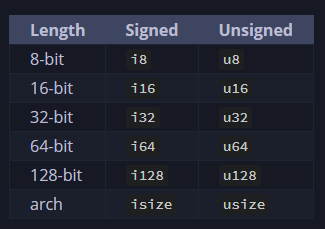The best place to start is the i32, as a default. isize or usize are mostly used for indexing collections.

Do keep in mind that overflows can happen, and if such a thing occurs, the program will panic at runtime (crash, in other words). Be mindful of the size of your variables!

Floating point numbers come in 2 varieties in Rust, and they represent numbers with decimal points. By default Rust uses f64, but the other version is f32. Today's CPUs are more capable and as such, the difference between using f64 and f32 is negligible, f64 beinc capable of more precision of course.

From the book..

``````fn main() {
let x = 2.0; // f64

let y: f32 = 3.0; // f32
}
``````

As for numeric operations, Rust supports all the expected ones. Addition, substraction, multiplication, division and remainder. Integer division rounds down to the closest integer.

Boolean type, with a size of 1 byte, can hold one of two values. True, or False. The type itself is written as bool.

``````fn main() {
let is_it_true: bool = true;

}
``````

Lastly, the char type which is for characters. Character literals are specified using single quotes, as opposed to double quotes which stand for string literals.

``````fn main() {
let some_character: char = 'Z';
}
``````

The Char type is a 4 byte value which can represent more than just ASCII. Accented letters, chinese, japanese characters, emojis, zero-space values. They are all valid for Rust's char type.

# Loops & Flow control

Rust has 3 options for loops. The classic for loop, while loop, and the loop loop. No it's not a typo.

``````fn main() {
loop {
println!("Hello there!");
}
}
``````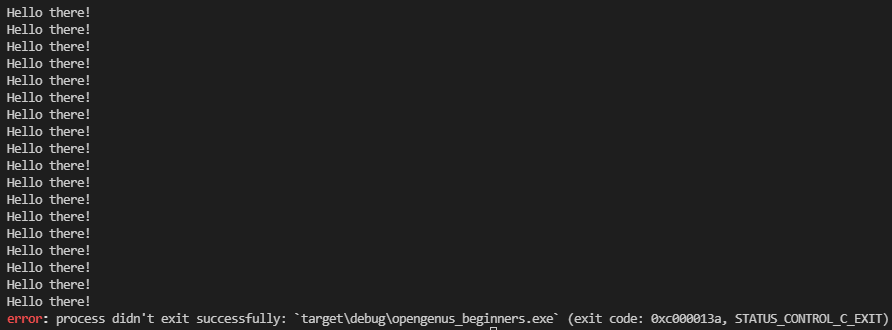As you can see, loop just..loops. Endlessly. The only way to stop it by killing it in the console, usually with ctrl + C command or equivalent in another platform. But what if we need to stop it mid-execution? That's where the break keyword comes in. For example..

``````fn main() {
let mut x = 0;

loop {
println!("X is: {}", x);
x += 1;

if x > 10 {
break;
}
}
}
``````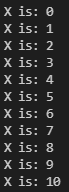Here I also introduce the if statement. After if, we write a statement that finally evaluates to either true or false. (Can also be a bool variable set somewhere else in the code). If it's true, it will enter the first set of brackets. If it's false, it either skips it, or enters the 'else' portion, written right after the closing brace.

The above code can be re-written this way, the output is exactly the same

``````fn main() {
let mut x = 0;

loop {
if x > 10 {
break;
} else {
println!("X is: {}", x);
x += 1;
}
}
}
``````

The while loop, loops until certain condition is broken. Rewriting our loop using a while loop looks like this:

``````fn main() {
let mut x = 0;

while x <= 10 {
println!("X is: {}", x);
x += 1;
}
}
``````

For loops take a variable, and use it to loop through an iterator. It works like a foreach in other languages.

``````fn main() {
for x in 0..11 { // 0..11 is syntax for creating a quick and dirty iterator.
println!("{}", x);
}
}
``````

# Functions

As most other programming languages if not all, Rust also has functions. In fact we've been seeing one all this time. The special main function. We can write our own functions by following the following syntax:

``````fn function_name(parameter_name: parameter_type, ..) -> return_type {}
``````

So for example, if we wanted to write a function that takes in a number, and prints it in a particular sentence, we'd write something like this:

``````fn main() {
print_a_number(42);
}

fn print_a_number(x: i32) {
println!("The number is: {}", x);
}
``````

As you can see, the return type is optional. Sometimes functions only need to do something, sometimes they need to work on some data and return a value. Like adding two numbers together..

``````fn main() {
}

fn add_two_numbers(x: i32, y: i32) -> i32 {
x + y
}
``````

Rust is special in that it generally does not need (but you can use) the return keyword when returning from a function. The only thing you have to remember is to not add the semicolon at the end of the returning statement. If we were to add a semicolon at the end of x + y we'd get an error, saying that the function returns () (AKA: Void, nothing), but we've specified it returns a i32 value type.

Comments are used for the programmers that read the code. Every comment is ignored by the compiler when it comes to running your program. They are typically used to explain the purpose of complicated functions or how they're supposed to work. Even to leave yourself little notes here and there so you don't forget to do certain things!

Rust has 3 ways of commenting like most other languages these days, shown below!

``````fn main() {
// The // allow you to add a line comment. It will only comment that line

/* For multiline
you use these sets of opening and closing
comment delimiters */
}

/// This generates DOC comments for the following item
/// This function adds two numbers and returns the result
/// The two numbers are of type i32, and so is the returned value
fn add_two_numbers(x: i32, y: i32) -> i32 {
x + y
}
``````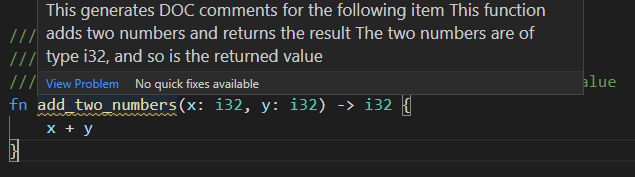# Arrays, Tuples and Vectors

A tuple is a way of grouping together several values into a single variable. They can be of different types. For example..

``````let my_typle : (i32, char, bool) = (42, 'l', true);
``````

This assigns that group of values to the variable "my_tuple". But how do we get the values out and use them?

``````fn main() {
let my_typle: (i32, char, bool) = (42, 'l', true);

println!(
"The first value is {}, second is {}, third is {}.",
my_typle.0, my_typle.1, my_typle.2
);

// Or you can use pattern matching to destructure the tuple, like so:

let (number, character, boolean) = my_typle;

println!(
"The number is {}, the character is {} and the boolean is {}",
number, character, boolean
);
}
``````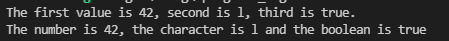Another way of storing multiple values in a single variable is the Array type. However this type is not as flexible as the Tuple. In Rust, array elements must all have the same type, and the type itself is fixed length. For example:

``````fn main() {
// Annotating the type for demonstration sake. The compiler can infer the type
// we need. The syntax is: [type; number of elements].
let my_array : [i32; 10] = [1, 2, 3, 4, 5, 6, 7, 8, 9, 10];

println!(
"The array is {} elements long and the 4th one is {}",
my_array.len(),
my_array
);
}
``````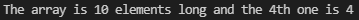This places those 10 numbers on the stack as opposed to the heap, as a single chunk of contiguous memory segments. If you don't know what any of that means, I've covered them in a previous article! So as you might probably guess, Arrays are great when you want to ensure that your data is stored on the stack, or if you want to ensure you always have a fixed number of elements. As you can see, since they are contiguous, they are indexed starting from 0 for the first element, all the way to n-1, n being the length of the array. If you attempt to access an index that is outside these bounds, the program will panic. You cannot read or write to memory that is not yours (yours as in, that variable's).

For a non-fixed length, growable type we have Vectors. They work the same way as Arrays, with a little bit of extra fluff! If we create an empty vector, we MUST add type annotation, otherwise the rust compiler can not know what type the elements are going to be. But as you can see, it's pretty much the same.

``````fn main() {
let my_vec: Vec<i32> = vec![1, 2, 3, 4, 5, 6, 7, 8, 9, 10];
let empty_vec: Vec<bool> = Vec::new();
println!(
"The array is {} elements long and the 4th one is {}",
my_vec.len(),
my_vec
);
}
``````

Vectors are growable and not fixed length. Meaning we can add and remove elements. For this we use push and pop respectively. But if we tried to push something into empty vec, it's gonna tell us we can't, because it's not mutable. Just because it can be grown, doesn't mean rust will let us without us explicitly stating so.

``````fn main() {
let mut empty_vec: Vec<bool> = Vec::new();

empty_vec.push(false);

if empty_vec.len() != 0 {
println!(
"Empty vec length is {}, but should be 0. Removing!",
empty_vec.len()
);
// Pop returns an option, because it might not always have something there. Thus we unwrap before using it.
let stored_value = empty_vec.pop().unwrap();
println!("{} was wrongfully stored in empty_vec", stored_value);

println!("Empty vec length is now: {}", empty_vec.len());
}
}
``````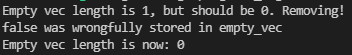Silly example. Also you might spot a problem. If Empty vec's length was bigger than 1, it would only remove one. We've already covered loops so.. how about you try to write a fix for this? :D Homework to practice!

# Strings

Strings are a bit of a complicated type in Rust. It's the first 'complex' type new rustaceans tend to run into. Strings are implemented as a collection of bytes. Unlike the previously discussed types, their size are not known at compile time. To make matters worse, there are 2 types of strings in Rust, 'str' and 'String'. 'str' is implemented directly into the core of the language, and is called a string slice, usually seen as '&str'. String itself is implemented in the standard library and is a growable, mutable, owned, UTF-8 encoded string type.

Strings are basically vectors of characters. They can be indexed the same way, have similar functions and are created the sameway.

``````fn main() {
let character_vector: Vec<char> = Vec::new();
let string: String = String::new();
}
``````

Although creating empty, immutable containers is not very useful. If we have some initial data for our string, we can use the ".to_string()" property on any type that implements the Display trait (or string literals). (Again, if you don't know traits, I've covered them in my first article!) There's also the String::from() method.

``````    let string: String = "This is a useful string. Indeed.".to_string();
let other_string: String = String::from("This is another useful string indeed");
``````

Strings are UTF-8 encoded, so all the following are valid strings (Taken from the book!)

``````let hello = String::from("السلام عليكم");
let hello = String::from("Dobrý den");
let hello = String::from("Hello");
let hello = String::from("שָׁלוֹם");
let hello = String::from("नमस्ते");
let hello = String::from("こんにちは");
let hello = String::from("안녕하세요");
let hello = String::from("你好");
let hello = String::from("Olá");
let hello = String::from("Здравствуйте");
let hello = String::from("Hola");
``````

Like vectors, you can push extra strings into a String, using the push_str function which works the same way as it does for vectors. You can also push single characters using only push(). Neat huh?

I advice you take a look at the strings chapter in the rust book because there are many details I'm not covering here, as this is just supposed to be an introduction!

# Type inference and casting

What do we mean when we say Type inference? Or like I've said all this time "The compiler can infer what type this is". I mean that the compiler can guess (most of the time), thanks to the stored values, what type the variable should be. Rust is a statically typed language, so all variables must have a type at compile time, either specified by us, or by the compiler's type inference capabilities.

Primitive types can be casted to each other although it can be a dangerous process. Rust has no implicit conversion, but you can do it explicitly with the 'as' keyword. I'll take the examples from the book, since they are better than anything I could possibly cook up.

There is a way of doing casting using the From and Into traits on custom types, but that I consider a more advanced topic. Just know it exists.

``````// Suppress all warnings from casts which overflow.
#![allow(overflowing_literals)]

fn main() {
let decimal = 65.4321_f32;

// Error! No implicit conversion
let integer: u8 = decimal;
// FIXME ^ Comment out this line

// Explicit conversion
let integer = decimal as u8;
let character = integer as char;

// Error! There are limitations in conversion rules.
// A float cannot be directly converted to a char.
let character = decimal as char;
// FIXME ^ Comment out this line

println!("Casting: {} -> {} -> {}", decimal, integer, character);

// when casting any value to an unsigned type, T,
// T::MAX + 1 is added or subtracted until the value
// fits into the new type

// 1000 already fits in a u16
println!("1000 as a u16 is: {}", 1000 as u16);

// 1000 - 256 - 256 - 256 = 232
// Under the hood, the first 8 least significant bits (LSB) are kept,
// while the rest towards the most significant bit (MSB) get truncated.
println!("1000 as a u8 is : {}", 1000 as u8);
// -1 + 256 = 255
println!("  -1 as a u8 is : {}", (-1i8) as u8);

// For positive numbers, this is the same as the modulus
println!("1000 mod 256 is : {}", 1000 % 256);

// When casting to a signed type, the (bitwise) result is the same as
// first casting to the corresponding unsigned type. If the most significant
// bit of that value is 1, then the value is negative.

// Unless it already fits, of course.
println!(" 128 as a i16 is: {}", 128 as i16);
// 128 as u8 -> 128, whose two's complement in eight bits is:
println!(" 128 as a i8 is : {}", 128 as i8);

// repeating the example above
// 1000 as u8 -> 232
println!("1000 as a u8 is : {}", 1000 as u8);
// and the two's complement of 232 is -24
println!(" 232 as a i8 is : {}", 232 as i8);

// Since Rust 1.45, the `as` keyword performs a *saturating cast*
// when casting from float to int. If the floating point value exceeds
// the upper bound or is less than the lower bound, the returned value
// will be equal to the bound crossed.

// 300.0 is 255
println!("300.0 is {}", 300.0_f32 as u8);
// -100.0 as u8 is 0
println!("-100.0 as u8 is {}", -100.0_f32 as u8);
// nan as u8 is 0
println!("nan as u8 is {}", f32::NAN as u8);

// This behavior incurs a small runtime cost and can be avoided
// with unsafe methods, however the results might overflow and
// return **unsound values**. Use these methods wisely:
unsafe {
// 300.0 is 44
println!("300.0 is {}", 300.0_f32.to_int_unchecked::<u8>());
// -100.0 as u8 is 156
println!("-100.0 as u8 is {}", (-100.0_f32).to_int_unchecked::<u8>());
// nan as u8 is 0
println!("nan as u8 is {}", f32::NAN.to_int_unchecked::<u8>());
}
}

``````

So this was a very high level overview of the bare basics of programming in Rust, as you know, practice makes permanent, so, go write some Rust code in your editor of choice and get some practice in! Happy coding!